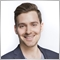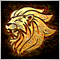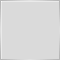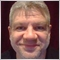# Really odd: EA function gets called before OnInit()...628

So this is odd. I have a function that is called before even OnInit() in my EA script. Of course this leads to problems...

Take a look. This is my initialize function:

[...]
double pips;

double BottomLevelPrice;
int LongTicketsStartLevel;
int ShortTicketsStartLevel;

int LongTickets[];
int ShortTickets[];

//+------------------------------------------------------------------+
//| Expert initialization function                                   |
//+------------------------------------------------------------------+
int OnInit()
{
Print("OnInit = ");
//---
double ticksize = MarketInfo(Symbol(), MODE_TICKSIZE);

if (ticksize == 0.00001 || ticksize == 0.001)
pips = ticksize*10;
else
pips = ticksize;
//---
// init first level
Print("ontick!");
BottomLevelPrice = MathFloor((Close-((double)InpLevelDistance/2*pips)) / pips) * pips; // to the nearest full pip
LongTicketsStartLevel = 0;
ShortTicketsStartLevel = 0;

return(INIT_SUCCEEDED);
}

And here is the one function that is (out of nowhere?) called even before OnInit():

int GetPriceLevel(double price)
{
Print("the pips is: ", pips, " bottom level price = ", BottomLevelPrice);
return (int)MathFloor((price-BottomLevelPrice) / (InpLevelDistance*pips));
}

So subsequently, the strategy tester journal looks like this:

PROBLEM:

When GetPriceLevel() is called (again, why??), obviously the local variables such as "pips" isn't initialized yet so it is zero, which results in an error. But why is this function even called before OnInit() in the first place? What am I missing? I'm really confused!Moderator
13664

It's probably in here:

[...]Moderator
2316

Try opening the Journal (right-click > view). Sometimes all the messages aren't printed in the tester window.

It is also not a good idea to access Close in OnInit()... that information might not be available yet.628

Marco vd Heijden:

It's probably in here:

[...]

Well it's just names and declerations. Here you go:

//+------------------------------------------------------------------+
//|                                             Leveled Hedge EA.mq4 |
//|                                                              Max |
//|                                             https://www.mql5.com |
//+------------------------------------------------------------------+
#property version   "1.00"
#property strict

extern int InpLevels=2; // Number of levels long / short
extern int InpTakeProfitLevels=2; // levels for take profit

extern int InpLevelDistance=30; // Level distance in pips

extern double LotSize = 0.01;
//---
extern int MagicNumber=3;

double pips;

double BottomLevelPrice;
int LongTicketsStartLevel;

[...]

honest_knave:

Try opening the Journal (right-click > view). Sometimes all the messages aren't printed in the tester window.

It is also not a good idea to access Close in OnInit()... that information might not be available yet.

Well I did that but it's exactly the same information as in the tester window as far as I'm  concerned.42167

Post ALL the code if you want help. Otherwise it's just a waste of time.Moderator
13664

It must be broken check { }25310

 RichPiano: When GetPriceLevel() is called You haven't posted any code that calls that, so we can't possibly help. There are no mind readers here - we can't see your code.The order of initialization is global and static variables, then OnInit. Do you have a class with a constructor that calls things?628

Alain Verleyen:

Post ALL the code if you want help. Otherwise it's just a waste of time.

I guess you're right so here we go:

//+------------------------------------------------------------------+
//|                                             Leveled Hedge EA.mq4 |
//|                                                              Max |
//|                                             https://www.mql5.com |
//+------------------------------------------------------------------+
#property version   "1.00"
#property strict

extern int InpLevels=2; // Number of levels long / short
extern int InpTakeProfitLevels=2; // levels for take profit

extern int InpLevelDistance=30; // Level distance in pips

extern double LotSize = 0.01;
//---
extern int MagicNumber=3;

double pips;

double BottomLevelPrice;
int LongTicketsStartLevel;
int ShortTicketsStartLevel;

int LongTickets[];
int ShortTickets[];

//+------------------------------------------------------------------+
//| Expert initialization function                                   |
//+------------------------------------------------------------------+
int OnInit()
{
Print("OnInit = ");
//---
double ticksize = MarketInfo(Symbol(), MODE_TICKSIZE);

if (ticksize == 0.00001 || ticksize == 0.001)
pips = ticksize*10;
else
pips = ticksize;
//---
// init first level
Print("ontick!");
BottomLevelPrice = MathFloor((Close-((double)InpLevelDistance/2*pips)) / pips) * pips; // to the nearest full pip
LongTicketsStartLevel = 0;
ShortTicketsStartLevel = 0;

return(INIT_SUCCEEDED);
}
//+------------------------------------------------------------------+
//| Expert deinitialization function                                 |
//+------------------------------------------------------------------+
void OnDeinit(const int reason)
{
//---

}
//+------------------------------------------------------------------+
//| Expert tick function                                             |
//+------------------------------------------------------------------+
void OnTick()
{
if (IsNewCandle())
}
//+------------------------------------------------------------------+

bool IsNewCandle()
{
static int BarsOnChart = 0;
if (BarsOnChart == Bars)
return (false);
BarsOnChart = Bars;
return (true);
}

{
static int prev_level = GetPriceLevel(Close);
int curr_level = GetPriceLevel(Close);
if (curr_level != prev_level)
{
// there must be hedged positions for each level
if (GetLongTicket(curr_level) < 0) OpenLongPosition();
if (GetShortTicket(curr_level) < 0) OpenShortPosition();

if (curr_level < prev_level) // buy low when price dropped
{
int pos = curr_level - ShortTicketsStartLevel;
// realize profits from positions that are in profit
for (int i = pos+InpTakeProfitLevels; i < ArraySize(ShortTickets); i++) // look above
CloseShortPosition(i);
// realize loss from positions that are too far away below
for (int i = pos-InpLevels; i >= 0; i--) // look below
CloseShortPosition(i);
}
else if (curr_level > prev_level) // sell high when price rises
{
int pos = curr_level - LongTicketsStartLevel;
// realize profits from positions that are in profit
for (int i = pos-InpTakeProfitLevels; i >= 0; i--) // look below
CloseLongPosition(i);
// realize loss from positions that are too far away above
for (int i = pos+InpLevels; i < ArraySize(ShortTickets); i++) // look above
CloseLongPosition(i);
}
}
prev_level = curr_level;
}

int OpenLongPosition()
{
Print("OpenLongPosition ");
double price = Ask; // always open at current price
int level = GetPriceLevel(price);

int ticket = OrderSend(Symbol(), OP_BUY, LotSize, price, 3, 0, 0, NULL, MagicNumber, 0, clrGreen);
if(ticket < 0)
{
Print("OrderSend failed with error #", GetLastError());
return -1;
}

// put in array
int size = ArraySize(LongTickets);
int pos = level - LongTicketsStartLevel;
if (pos < 0)
{
ArrayResize(LongTickets, size-pos, 10); // since pos is negative
for (int i=size-pos-1; i >= 0; i--)
{
if (i+pos < 0)
LongTickets[i] = -1; // fill front with -1
else
LongTickets[i] = LongTickets[i+pos];
}
LongTicketsStartLevel = level; // new ground zero
}
else if (pos >= size)
{
ArrayResize(LongTickets, pos+1, 10);
// fill back with -1 (no ticket)
for (int i=size; i < pos+1; i++)
LongTickets[i] = -1;
}
LongTickets[pos] = ticket;
return true;
}

//--- OP_SELL

int OpenShortPosition()
{
Print("OpenShortPosition ");
double price = Bid; // always open at current price
int level = GetPriceLevel(price);

int ticket = OrderSend(Symbol(), OP_SELL, LotSize, price, 3, 0, 0, NULL, MagicNumber, 0, clrRed);
if(ticket < 0)
{
Print("OrderSend failed with error #", GetLastError());
return -1;
}

// put in array
int size = ArraySize(ShortTickets);
int pos = level - ShortTicketsStartLevel;
if (pos < 0)
{
ArrayResize(ShortTickets, size-pos, 10); // since pos is negative
for (int i=size-pos-1; i >= 0; i--)
{
if (i+pos < 0)
ShortTickets[i] = -1; // fill front with -1
else
ShortTickets[i] = ShortTickets[i+pos];
}
ShortTicketsStartLevel = level; // new ground zero
}
else if (pos >= size)
{
ArrayResize(ShortTickets, pos+1, 10);
// fill back with -1 (no ticket)
for (int i=size; i < pos+1; i++)
ShortTickets[i] = -1;
}
ShortTickets[pos] = ticket;
return true;
}

//--- ticket

int GetLongTicket(int level)
{
int pos = level - LongTicketsStartLevel;
if (pos < 0 || pos > ArraySize(LongTickets)-1)
return -1;
else
return LongTickets[pos];
}

int GetShortTicket(int level)
{
int pos = level - ShortTicketsStartLevel;
if (pos < 0 || pos > ArraySize(ShortTickets)-1)
return -1;
else
return ShortTickets[pos];
}

//--- close

bool CloseLongPosition(int pos)
{
int ticket = LongTickets[pos];

if (OrderClose(ticket, LotSize, Bid, 3, clrRed)) // sell
{
LongTickets[pos] = -1;
return true;
}
return false;
}

bool CloseShortPosition(int pos)
{
int ticket = ShortTickets[pos];

{
ShortTickets[pos] = -1;
return true;
}
return false;
}

int GetPriceLevel(double price)
{
Print("the pips is: ", pips, " bottom level price = ", BottomLevelPrice);
return (int)MathFloor((price-BottomLevelPrice) / (InpLevelDistance*pips));
}

whroeder1:
 You haven't posted any code that calls that, so we can't possibly help. There are no mind readers here - we can't see your code.The order of initialization is global and static variables, then OnInit. Do you have a class with a constructor that calls things?

The function is only called on four occasions, but none of these happen before OnInit(). I checked it. This is what leaves me clueless. The script also doesn't use any classes.42167

RichPiano:

I guess you're right so here we go:

...

The function is only called on four occasions, but none of these happen before OnInit(). I checked it. This is what leaves me clueless. The script also doesn't use any classes.

{
static int prev_level = GetPriceLevel(Close);
int curr_level = GetPriceLevel(Close);
...
}
A static is initialized BEFORE OnInit() so your GetPriceLevel() function is called, your message "the pips is..." is printed and you get a Zero Divide error as "pips" is 0.
Static variables exist from the moment of program execution and are initialized only once before the specialized functions OnInit() is called. If the initial values are not specified, variables of the static storage class are taking zero initial values.
Also you are using Close before OnInit() so you will get sooner or later an Array Out of Range error too.Moderator
13664

{
static int prev_level = GetPriceLevel(Close);
int curr_level = GetPriceLevel(Close);
if (curr_level != prev_level)
{
// there must be hedged positions for each level
if (GetLongTicket(curr_level) < 0) OpenLongPosition();
if (GetShortTicket(curr_level) < 0) OpenShortPosition();

if (curr_level < prev_level) // buy low when price dropped
{
int pos = curr_level - ShortTicketsStartLevel;
// realize profits from positions that are in profit
for (int i = pos+InpTakeProfitLevels; i < ArraySize(ShortTickets); i++) // look above
CloseShortPosition(i);
// realize loss from positions that are too far away below
for (int i = pos-InpLevels; i >= 0; i--) // look below
CloseShortPosition(i);
}
else if (curr_level > prev_level) // sell high when price rises
{
int pos = curr_level - LongTicketsStartLevel;
// realize profits from positions that are in profit
for (int i = pos-InpTakeProfitLevels; i >= 0; i--) // look below
CloseLongPosition(i);
// realize loss from positions that are too far away above
for (int i = pos+InpLevels; i < ArraySize(ShortTickets); i++) // look above
CloseLongPosition(i);
}
}
prev_level = curr_level;
}
You can not declare a static integer and then further on try to adjust it's value.Moderator
13664

Ah i was too late ;)

Anyway try this:

{
int prev_level = GetPriceLevel(Close);
int curr_level = GetPriceLevel(Close);
if (curr_level != prev_level)
{
// there must be hedged positions for each level
if (GetLongTicket(curr_level) < 0) OpenLongPosition();
if (GetShortTicket(curr_level) < 0) OpenShortPosition();

if (curr_level < prev_level) // buy low when price dropped
{
int pos = curr_level - ShortTicketsStartLevel;
// realize profits from positions that are in profit
for (int i = pos+InpTakeProfitLevels; i < ArraySize(ShortTickets); i++) // look above
CloseShortPosition(i);
// realize loss from positions that are too far away below
for (int i = pos-InpLevels; i >= 0; i--) // look below
CloseShortPosition(i);
}
else if (curr_level > prev_level) // sell high when price rises
{
int pos = curr_level - LongTicketsStartLevel;
// realize profits from positions that are in profit
for (int i = pos-InpTakeProfitLevels; i >= 0; i--) // look below
CloseLongPosition(i);
// realize loss from positions that are too far away above
for (int i = pos+InpLevels; i < ArraySize(ShortTickets); i++) // look above
CloseLongPosition(i);
}
}
prev_level = curr_level;
}